## 10

The number 10 (ten) is the basis for the Decimal system of notation. In this system, each decimal place'' consists of a Digit 0-9 arranged such that each Digit is multiplied by a Power of 10, decreasing from left to right, and with a decimal place indicating the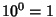s place. For example, the number 1234.56 specifiesThe decimal places to the left of the decimal point are 1, 10, 100, 1000, 10000, 100000, 1000000, 10000000, 100000000, ... (Sloane's A011557), called one, ten, Hundred, Thousand, ten thousand, hundred thousand, Million, 10 million, 100 million, and so on. The names of subsequent decimal places for Large Numbers differ depending on country.

Any Power of 10 which can be written as the Product of two numbers not containing 0s must be of the formforan Integer such that neithernorcontains any Zeros. The largest known such number is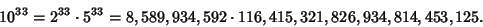A complete list of known such numbers is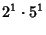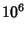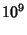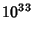(Madachy 1979). Since all Powers of 2 with exponentscontain at least one Zero (Cook), no other Power of ten less than 46 million can be written as the Product of two numbers not containing 0s.

See also Billion, Decimal, Hundred, Large Number, Milliard, Million, Thousand, Trillion, Zero

References

Madachy, J. S. Madachy's Mathematical Recreations. New York: Dover, pp. 127-128, 1979.

Pickover, C. A. Keys to Infinity. New York: Wiley, p. 135, 1995.

Sloane, N. J. A. Sequence A011557 in The On-Line Version of the Encyclopedia of Integer Sequences.'' http://www.research.att.com/~njas/sequences/eisonline.html.